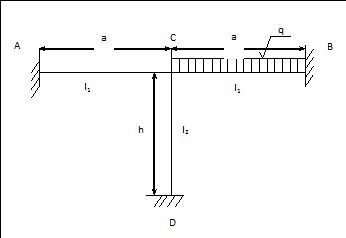# Rigid frame calculation

I need assistance with below problem:

Known are: a, h, I1, I2, q. Problem find moment at C. The beams are rigidly connected at "C"!Apparently the right solution is:

Mc=q*a^2/12 * 1 / (1+2*h*I1/a*I2), while mine solution is Mc=q*a^2/12 * 1 / (1+h*I1/a*I2).

I have used superposition method, cut the frame at "C", add moment Mc, and equating the slopes of the vertical beam CD and loaded portion of the horizontal beam AB that is CB.

Regards

F.

### Re: Rigid frame calculation

... Anybody have idea? Thanks

• Mathematics inside the configured delimiters is rendered by MathJax. The default math delimiters are $$...$$ and $...$ for displayed mathematics, and $...$ and $...$ for in-line mathematics.